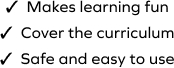# Find the Remainder When Dividing a 3-Digit Number by 1-Digit Number

SplashLearn - The complete PreK - Grade 5 Math & ELA Learning Program Built for Your Child
Home > Find the Remainder When Dividing a 3-Digit Number by 1-Digit Number
The game encourages your young mathematician to use their understanding of division to find the answer. The student will divide 3-digit numbers by 1-digit numbers to find the remainder in this game. Students will need to analyze and select the correct answer from a set of given options.